# How do we know whether the 2 and the 2's match up as in the explanation and whether the a's match up also.

• Module 2 Week 3 Day 12 Challenge Part 2

Whether the 2 and the 2's match up as in the explanation and whether the a's match up also.

• @TSS-Graviser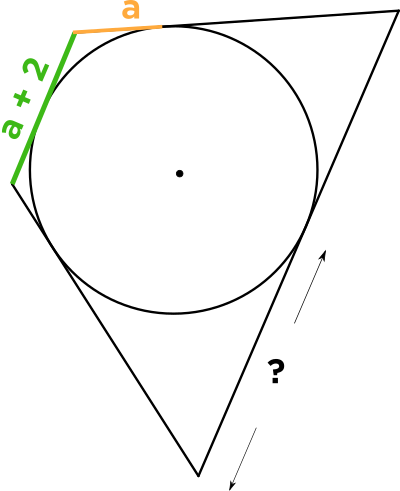This is a good question! There are a lot of behind-the-scenes ideas in this problem. The first piece of the puzzle is that from the tangent points of the quadrilateral and the circle to the vertex between them, these two segments are the same length. These would be the yellow segments in the diagram. Why is this? Well, it's because you can see that there are two similar right triangles which have this yellow segment as their short leg.

The right angle derives from the tangent line being perpendicular to a radius, and the other legs are both radii, so they are the same length. Since they share the same hypotenuse in the middle, these triangles are in fact congruent! Thus the yellow segments are the same length.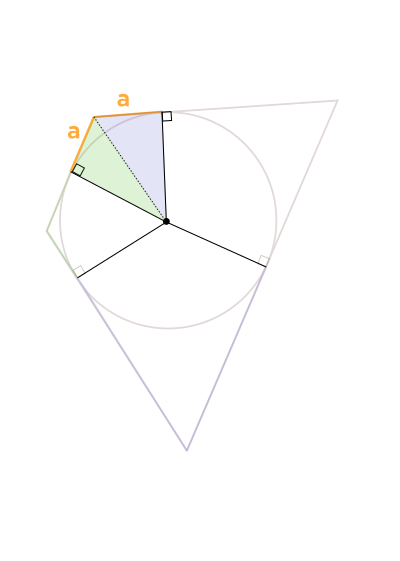Working our way around, we can do the same thing for the next set of congruent right triangles, and find that the two green segments are the same length. Since to get the length of this green segment, we subtract out the \(a\) segment from the longer \(a + 2\) segment, the length of this segment is \(2.\)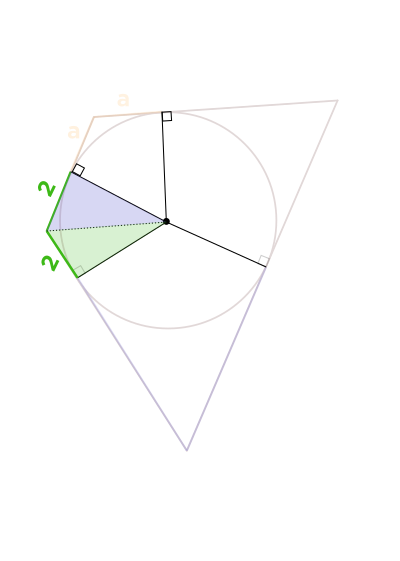The last set of congruent triangles shows us that the missing length of the purple segment on the right must be equal to \(5:\)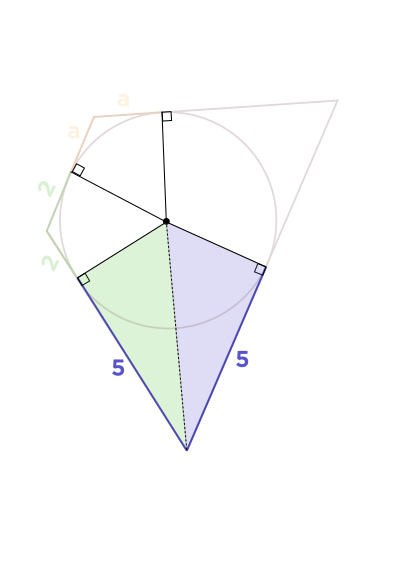Putting it all together, we now know all of the unknown lengths from our diagram: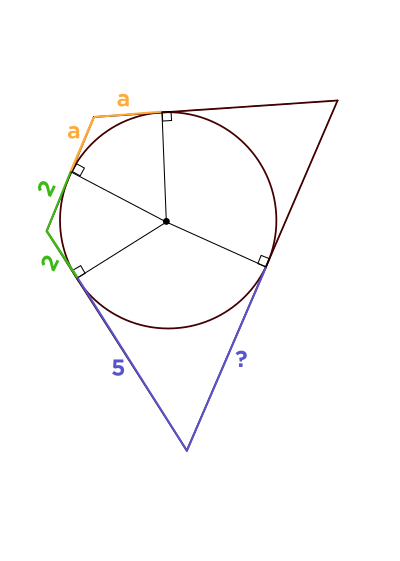The name for this double-congruent-right-triangle is "kite," and so it's convenient to remember that from now on, whenever you see a quadrilateral with an inscribed circle, you will always be able to piece apart the quadrilateral into four kites. And kites have two pairs of equal-length sides.